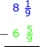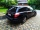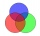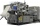# Fraction calculator

The calculator performs basic and advanced operations with fractions, expressions with fractions combined with integers, decimals, and mixed numbers. It also shows detailed step-by-step information about the fraction calculation procedure. Solve problems with two, three, or more fractions and numbers in one expression.

## Result:

### 86/10 - 47/12 = 241/60 = 4 1/60 ≅ 4.0166667

Spelled result in words is two hundred forty-one sixtieths (or four and one sixtieth).

### How do you solve fractions step by step?

1. Conversion a mixed number 8 6/10 to a improper fraction: 8 6/10 = 8 6/10 = 8 · 10 + 6/10 = 80 + 6/10 = 86/10

To find a new numerator:
a) Multiply the whole number 8 by the denominator 10. Whole number 8 equally 8 * 10/10 = 80/10
b) Add the answer from previous step 80 to the numerator 6. New numerator is 80 + 6 = 86
c) Write a previous answer (new numerator 86) over the denominator 10.

Eight and six tenths is eighty-six tenths
2. Conversion a mixed number 4 7/12 to a improper fraction: 4 7/12 = 4 7/12 = 4 · 12 + 7/12 = 48 + 7/12 = 55/12

To find a new numerator:
a) Multiply the whole number 4 by the denominator 12. Whole number 4 equally 4 * 12/12 = 48/12
b) Add the answer from previous step 48 to the numerator 7. New numerator is 48 + 7 = 55
c) Write a previous answer (new numerator 55) over the denominator 12.

Four and seven twelfths is fifty-five twelfths
3. Subtract: 86/10 - 55/12 = 86 · 6/10 · 6 - 55 · 5/12 · 5 = 516/60 - 275/60 = 516 - 275/60 = 241/60
For adding, subtracting, and comparing fractions, it is suitable to adjust both fractions to a common (equal, identical) denominator. The common denominator you can calculate as the least common multiple of both denominators - LCM(10, 12) = 60. In practice, it is enough to find the common denominator (not necessarily the lowest) by multiplying the denominators: 10 × 12 = 120. In the following intermediate step, it cannot further simplify the fraction result by canceling.
In other words - eighty-six tenths minus fifty-five twelfths = two hundred forty-one sixtieths.

#### Rules for expressions with fractions:

Fractions - simply use a forward slash between the numerator and denominator, i.e., for five-hundredths, enter 5/100. If you are using mixed numbers, be sure to leave a single space between the whole and fraction part.
The slash separates the numerator (number above a fraction line) and denominator (number below).

Mixed numerals (mixed fractions or mixed numbers) write as integer separated by one space and fraction i.e., 1 2/3 (having the same sign). An example of a negative mixed fraction: -5 1/2.
Because slash is both signs for fraction line and division, we recommended use colon (:) as the operator of division fractions i.e., 1/2 : 3.

Decimals (decimal numbers) enter with a decimal point . and they are automatically converted to fractions - i.e. 1.45.

The colon : and slash / is the symbol of division. Can be used to divide mixed numbers 1 2/3 : 4 3/8 or can be used for write complex fractions i.e. 1/2 : 1/3.
An asterisk * or × is the symbol for multiplication.
Plus + is addition, minus sign - is subtraction and ()[] is mathematical parentheses.
The exponentiation/power symbol is ^ - for example: (7/8-4/5)^2 = (7/8-4/5)2

#### Examples:

subtracting fractions: 2/3 - 1/2
multiplying fractions: 7/8 * 3/9
dividing Fractions: 1/2 : 3/4
exponentiation of fraction: 3/5^3
fractional exponents: 16 ^ 1/2
adding fractions and mixed numbers: 8/5 + 6 2/7
dividing integer and fraction: 5 ÷ 1/2
complex fractions: 5/8 : 2 2/3
decimal to fraction: 0.625
Fraction to Decimal: 1/4
Fraction to Percent: 1/8 %
comparing fractions: 1/4 2/3
multiplying a fraction by a whole number: 6 * 3/4
square root of a fraction: sqrt(1/16)
reducing or simplifying the fraction (simplification) - dividing the numerator and denominator of a fraction by the same non-zero number - equivalent fraction: 4/22
expression with brackets: 1/3 * (1/2 - 3 3/8)
compound fraction: 3/4 of 5/7
fractions multiple: 2/3 of 3/5
divide to find the quotient: 3/5 ÷ 2/3

The calculator follows well-known rules for order of operations. The most common mnemonics for remembering this order of operations are:
PEMDAS - Parentheses, Exponents, Multiplication, Division, Addition, Subtraction.
BEDMAS - Brackets, Exponents, Division, Multiplication, Addition, Subtraction
BODMAS - Brackets, Of or Order, Division, Multiplication, Addition, Subtraction.
GEMDAS - Grouping Symbols - brackets (){}, Exponents, Multiplication, Division, Addition, Subtraction.
Be careful, always do multiplication and division before addition and subtraction. Some operators (+ and -) and (* and /) has the same priority and then must evaluate from left to right.

## Fractions in word problems:

• CupcakesIn a bowl was some cupcakes. Janka ate one third and Danka ate one quarter of cupcakes. a) How many of cookies ate together? b) How many cookies remain in a bowl? Write the results as a decimal number and in notepad also as a fraction.
• Square metal sheetWe cut out four squares of 300 mm side from a square sheet metal plate with a side of 0,7 m. Express the fraction and the percentage of waste from the square metal sheet.
• ShopperEva spent 1/4 in one store and 1/3 in another. What fraction is left?
• Peter's calculationPeter wrote the following: 7 1/4 - 3 3/4 = 4 2/4 = 4 1/2 . Is Peter’s calculation correct? Using words (math vocabulary) and numbers explain why he is correct or incorrect.
• Evaluate - lowest termsEvaluate: 16/25 - 11/25 (Express answer as a fraction reduced to lowest terms. )
• Difference of two fractionsWhat is the difference between 1/2 and 1/6? (Write the answer as a fraction in lowest terms. )
• Coloured teacupsThe teacups in Tea Stop 55 are `2/5` green and `3/10` yellow. What fraction of the teacups are neither green nor yellow?
• A marketA market vendor was able to sell all the mangoes, papayas, and star apples.  1/5  of the fruits were mangoes,   2/3  of them were papayas and the rest were star apples.  How many parts of the fruits sold are star apples?
• Circular gardenAlice creates a circular vegetable garden. Tomatoes are planted in 1/3 of the circular garden, carrots are planted in 2/5 of the circular garden, and green peppers are planted in 1/10 of the circular garden. What fraction represents the remaining unplante
• SarahSarah has a photography blog. 3/7 of her photos are of nature, 1/4 of the rest are of her friends. What fraction of all of Sarah's photos is of her friends?
• A laundryMr. Green washed 1/4 of his laundry. His son washed 3/7 of it. Who washed most of the laundry? How much of the laundry still needs to be washed?
• Difference mixed fractionsWhat is the difference between 4 2/3 and 3 1/6?Erika’s career consists of filing, typing and answering phones. She spends 1/6 of her time filing and 5/8 of her time typing. What fraction of her time does she spend answering phone calls?